# Photon

Photon
Composition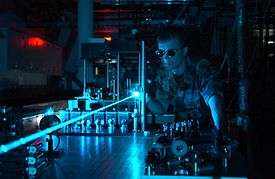Photons emitted in a coherent beam from a laser Elementary particle Bosonic Electromagnetic γ, hν, or ħω Albert Einstein 0 <1×10−18 eV/c2 Stable 0 <1×10−35 e 1 -1 -1 I(JPC) = 0,1(1--)

In physics, a photon is an elementary particle, the quantum of the electromagnetic interaction and the basic unit of light and all other forms of electromagnetic radiation. It is also the force carrier for the electromagnetic force. The effects of this force are easily observable at both the microscopic and macroscopic level, because the photon has no rest mass; this allows for interactions at long distances. Like all elementary particles, photons are currently best explained by quantum mechanics and will exhibit wave–particle duality, exhibiting properties of both waves and particles. For example, a single photon may be refracted by a lens or exhibit wave interference with itself, but also act as a particle giving a definite result when quantitative momentum (quantized angular momentum) is measured.

The modern concept of the photon was developed gradually by Albert Einstein to explain experimental observations that did not fit the classical wave model of light. In particular, the photon model accounted for the frequency dependence of light's energy, and explained the ability of matter and radiation to be in thermal equilibrium. It also accounted for anomalous observations, including the properties of black body radiation, that other physicists, most notably Max Planck, had sought to explain using semiclassical models, in which light is still described by Maxwell's equations, but the material objects that emit and absorb light are quantized. Although these semiclassical models contributed to the development of quantum mechanics, further experiments validated Einstein's hypothesis that light itself is quantized; the quanta of light are photons.

In the Standard Model of particle physics, photons are described as a necessary consequence of physical laws having a certain symmetry at every point in spacetime. The intrinsic properties of photons, such as charge, mass and spin, are determined by the properties of this gauge symmetry. The neutrino theory of light, which attempts to describe the photon as a composite structure, has been unsuccessful so far.

The photon concept has led to momentous advances in experimental and theoretical physics, such as lasers, Bose–Einstein condensation, quantum field theory, and the probabilistic interpretation of quantum mechanics. It has been applied to photochemistry, high-resolution microscopy, and measurements of molecular distances. Recently, photons have been studied as elements of quantum computers and for sophisticated applications in optical communication such as quantum cryptography.

## Nomenclature

In 1900, Max Planck was working on black-body radiation and suggested that the energy in electromagnetic waves could only be released in "packets" of energy. In his 1901 article  in Annalen der Physik he called these packets "energy elements". The word quanta (singular quantum) was used even before 1900 to mean particles or amounts of different quantities, including electricity. Later, in 1905 Albert Einstein went further by suggesting that electromagnetic waves could only exist in these discrete wave-packets. He called such a wave-packet the light quantum (German: das Lichtquant). The name photon derives from the Greek word for light, φως (transliterated phôs), and was coined[Note 1] in 1926 by the physical chemist Gilbert Lewis, who published a speculative theory in which photons were "uncreatable and indestructible". Although Lewis' theory was never accepted as it was contradicted by many experiments, his new name, photon, was adopted immediately by most physicists. Isaac Asimov credits Arthur Compton with defining quanta of energy as photons in 1923.

In physics, a photon is usually denoted by the symbol γ (the Greek letter gamma). This symbol for the photon probably derives from gamma rays, which were discovered in 1900 by Paul Villard, named by Ernest Rutherford in 1903, and shown to be a form of electromagnetic radiation in 1914 by Rutherford and Edward Andrade. In chemistry and optical engineering, photons are usually symbolized by , the energy of a photon, where h is Planck's constant and the Greek letter ν (nu) is the photon's frequency. Much less commonly, the photon can be symbolized by hf, where its frequency is denoted by f.

## Physical properties

The photon is massless,[Note 2] has no electric charge, and does not decay spontaneously in empty space[citation needed]. A photon has two possible polarization states and is described by exactly three continuous parameters: the components of its wave vector, which determine its wavelength λ and its direction of propagation. The photon is the gauge boson for electromagnetism, and therefore all other quantum numbers of the photon (such as lepton number, baryon number, and flavour quantum numbers) are zero.

Photons are emitted in many natural processes. For example, when a charge is accelerated it emits synchrotron radiation. During a molecular, atomic or nuclear transition to a lower energy level, photons of various energy will be emitted, from infrared light to gamma rays. A photon can also be emitted when a particle and its corresponding antiparticle are annihilated (for example, electron-positron annihilation).

In empty space, the photon moves at c (the speed of light) and its energy and momentum are related by E = pc, where p is the magnitude of the momentum vector p. This derives from the following relativistic relation, with m = 0:

E2 = p2c2 + m2c4.

The energy and momentum of a photon depend only on its frequency (ν) or inversely, its wavelength (λ):$E = \hbar\omega = h\nu = \frac{h c}{\lambda}$$\mathbf{p} = \hbar\mathbf{k},$

where k is the wave vector (where the wave number k = |k| = 2π/λ), ω = 2πν is the angular frequency, and ħ = h/2π is the reduced Planck constant.

Since p points in the direction of the photon's propagation, the magnitude of the momentum is$p = \hbar k = \frac{h\nu}{c} = \frac{h}{\lambda}.$

The photon also carries spin angular momentum that does not depend on its frequency. The magnitude of its spin is$\scriptstyle{\sqrt{2} \hbar}$ and the component measured along its direction of motion, its helicity, must be ±ħ. These two possible helicities, called right-handed and left-handed, correspond to the two possible circular polarization states of the photon.

To illustrate the significance of these formulae, the annihilation of a particle with its antiparticle in free space must result in the creation of at least two photons for the following reason. In the center of mass frame, the colliding antiparticles have no net momentum, whereas a single photon always has momentum (since it is determined, as we have seen, only by the photon's frequency or wavelength—which cannot be zero). Hence, conservation of momentum (or equivalently, translational invariance) requires that at least two photons are created, with zero net momentum. (However, it is possible if the system interacts with another particle or field for annihilation to produce one photon, as when a positron annihilates with a bound atomic electron, it is possible for only one photon to be emitted, as the nuclear Coulomb field breaks translational symmetry.) The energy of the two photons, or, equivalently, their frequency, may be determined from conservation of four-momentum. Seen another way, the photon can be considered as its own antiparticle. The reverse process, pair production, is the dominant mechanism by which high-energy photons such as gamma rays lose energy while passing through matter. That process is the reverse of "annihilation to one photon" allowed in the electric field of an atomic nucleus.

The classical formulae for the energy and momentum of electromagnetic radiation can be re-expressed in terms of photon events. For example, the pressure of electromagnetic radiation on an object derives from the transfer of photon momentum per unit time and unit area to that object, since pressure is force per unit area and force is the change in momentum per unit time.

### Experimental checks on photon mass

The photon is currently understood to be strictly massless, but this is an experimental question. If the photon is not a strictly massless particle, it would not move at the exact speed of light in vacuum, c. Its speed would be lower and depend on its frequency. Relativity would be unaffected by this; the so-called speed of light, c, would then not be the actual speed at which light moves, but a constant of nature which is the maximum speed that any object could theoretically attain in space-time. Thus, it would still be the speed of space-time ripples (gravitational waves and gravitons), but it would not be the speed of photons.

A massive photon would have other effects as well. Coulomb's law would be modified and the electromagnetic field would have an extra physical degree of freedom. These effects yield more sensitive experimental probes of the photon mass than the frequency dependence of the speed of light. If Coulomb's law is not exactly valid, then that would cause the presence of an electric field inside a hollow conductor when it is subjected to an external electric field. This thus allows one to test Coulomb's law to very high precision. A null result of such an experiment has set a limit of m ≲ 10−14 eV/c2.

Sharper upper limits have been obtained in experiments designed to detect effects caused by the Galactic vector potential. Although the galactic vector potential is very large because the galactic magnetic field exists on very long length scales, only the magnetic field is observable if the photon is massless. In case of a massive photon, the mass term$\scriptstyle\frac{1}{2} m^2 A_{\mu}A^{\mu}$ would affect the galactic plasma. The fact that no such effects are seen implies an upper bound on the photon mass of m < 3×10−27 eV/c2. The galactic vector potential can also be probed directly by measuring the torque exerted on a magnetized ring. Such methods were used to obtain the sharper upper limit of 10−18eV/c2 given by the Particle Data Group.

These sharp limits from the non-observation of the effects caused by the galactic vector potential have been shown to be model dependent. If the photon mass is generated via the Higgs mechanism then the upper limit of m≲10−14 eV/c2 from the test of Coulomb's law is valid.

Photons inside superconductors do develop a nonzero effective rest mass; as a result, electromagnetic forces become short-range inside superconductors.

## Historical development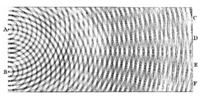Thomas Young's double-slit experiment in 1805 showed that light can act as a wave, helping to defeat early particle theories of light.

In most theories up to the eighteenth century, light was pictured as being made up of particles. Since particle models cannot easily account for the refraction, diffraction and birefringence of light, wave theories of light were proposed by René Descartes (1637), Robert Hooke (1665), and Christian Huygens (1678); however, particle models remained dominant, chiefly due to the influence of Isaac Newton. In the early nineteenth century, Thomas Young and August Fresnel clearly demonstrated the interference and diffraction of light and by 1850 wave models were generally accepted. In 1865, James Clerk Maxwell's prediction that light was an electromagnetic wave—which was confirmed experimentally in 1888 by Heinrich Hertz's detection of radio waves—seemed to be the final blow to particle models of light.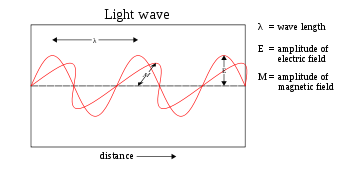In 1900, Maxwell's theoretical model of light as oscillating electric and magnetic fields seemed complete. However, several observations could not be explained by any wave model of electromagnetic radiation, leading to the idea that light-energy was packaged into quanta described by E=hν. Later experiments showed that these light-quanta also carry momentum and, thus, can be considered particles: the photon concept was born, leading to a deeper understanding of the electric and magnetic fields themselves.

The Maxwell wave theory, however, does not account for all properties of light. The Maxwell theory predicts that the energy of a light wave depends only on its intensity, not on its frequency; nevertheless, several independent types of experiments show that the energy imparted by light to atoms depends only on the light's frequency, not on its intensity. For example, some chemical reactions are provoked only by light of frequency higher than a certain threshold; light of frequency lower than the threshold, no matter how intense, does not initiate the reaction. Similarly, electrons can be ejected from a metal plate by shining light of sufficiently high frequency on it (the photoelectric effect); the energy of the ejected electron is related only to the light's frequency, not to its intensity.[Note 3]

At the same time, investigations of blackbody radiation carried out over four decades (1860–1900) by various researchers culminated in Max Planck's hypothesis that the energy of any system that absorbs or emits electromagnetic radiation of frequency ν is an integer multiple of an energy quantum E=hν. As shown by Albert Einstein, some form of energy quantization must be assumed to account for the thermal equilibrium observed between matter and electromagnetic radiation; for this explanation of the photoelectric effect, Einstein received the 1921 Nobel Prize in physics.

Since the Maxwell theory of light allows for all possible energies of electromagnetic radiation, most physicists assumed initially that the energy quantization resulted from some unknown constraint on the matter that absorbs or emits the radiation. In 1905, Einstein was the first to propose that energy quantization was a property of electromagnetic radiation itself. Although he accepted the validity of Maxwell's theory, Einstein pointed out that many anomalous experiments could be explained if the energy of a Maxwellian light wave were localized into point-like quanta that move independently of one another, even if the wave itself is spread continuously over space. In 1909 and 1916, Einstein showed that, if Planck's law of black-body radiation is accepted, the energy quanta must also carry momentum p=h/λ, making them full-fledged particles. This photon momentum was observed experimentally by Arthur Compton, for which he received the Nobel Prize in 1927. The pivotal question was then: how to unify Maxwell's wave theory of light with its experimentally observed particle nature? The answer to this question occupied Albert Einstein for the rest of his life, and was solved in quantum electrodynamics and its successor, the Standard Model (see Second quantization and The photon as a gauge boson, below).

## Early objections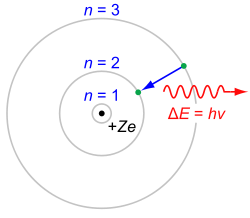Up to 1923, most physicists were reluctant to accept that light itself was quantized. Instead, they tried to explain photon behavior by quantizing only matter, as in the Bohr model of the hydrogen atom (shown here). Even though these semiclassical models were only a first approximation, they were accurate for simple systems and they led to quantum mechanics.

Einstein's 1905 predictions were verified experimentally in several ways in the first two decades of the 20th century, as recounted in Robert Millikan's Nobel lecture. However, before Compton's experiment showing that photons carried momentum proportional to their wave number (or frequency) (1922), most physicists were reluctant to believe that electromagnetic radiation itself might be particulate. (See, for example, the Nobel lectures of Wien, Planck and Millikan.). Instead, there was a widespread belief that energy quantization resulted from some unknown constraint on the matter that absorbs or emits radiation. Attitudes changed over time. In part, the change can be traced to experiments such as Compton scattering, where it was much more difficult not to ascribe quantization to light itself to explain the observed results.

Even after Compton's experiment, Niels Bohr, Hendrik Kramers and John Slater made one last attempt to preserve the Maxwellian continuous electromagnetic field model of light, the so-called BKS model. To account for the then-available data, two drastic hypotheses had to be made:

1. Energy and momentum are conserved only on the average in interactions between matter and radiation, not in elementary processes such as absorption and emission. This allows one to reconcile the discontinuously changing energy of the atom (jump between energy states) with the continuous release of energy into radiation.
2. Causality is abandoned. For example, spontaneous emissions are merely emissions induced by a "virtual" electromagnetic field.

However, refined Compton experiments showed that energy-momentum is conserved extraordinarily well in elementary processes; and also that the jolting of the electron and the generation of a new photon in Compton scattering obey causality to within 10 ps. Accordingly, Bohr and his co-workers gave their model "as honorable a funeral as possible". Nevertheless, the failures of the BKS model inspired Werner Heisenberg in his development of matrix mechanics.

A few physicists persisted in developing semiclassical models in which electromagnetic radiation is not quantized, but matter appears to obey the laws of quantum mechanics. Although the evidence for photons from chemical and physical experiments was overwhelming by the 1970s, this evidence could not be considered as absolutely definitive; since it relied on the interaction of light with matter, a sufficiently complicated theory of matter could in principle account for the evidence. Nevertheless, all semiclassical theories were refuted definitively in the 1970s and 1980s by photon-correlation experiments.[Note 4] Hence, Einstein's hypothesis that quantization is a property of light itself is considered to be proven.

## Wave–particle duality and uncertainty principles

Photons, like all quantum objects, exhibit both wave-like and particle-like properties. Their dual wave–particle nature can be difficult to visualize. The photon displays clearly wave-like phenomena such as diffraction and interference on the length scale of its wavelength. For example, a single photon passing through a double-slit experiment lands on the screen exhibiting interference phenomena but only if no measure was made on the actual slit being run across. To account for the particle interpretation that phenomenon is called probability distribution but behaves according to the Maxwell's equations. However, experiments confirm that the photon is not a short pulse of electromagnetic radiation; it does not spread out as it propagates, nor does it divide when it encounters a beam splitter. Rather, the photon seems to be a point-like particle since it is absorbed or emitted as a whole by arbitrarily small systems, systems much smaller than its wavelength, such as an atomic nucleus (≈10−15 m across) or even the point-like electron. Nevertheless, the photon is not a point-like particle whose trajectory is shaped probabilistically by the electromagnetic field, as conceived by Einstein and others; that hypothesis was also refuted by the photon-correlation experiments cited above. According to our present understanding, the electromagnetic field itself is produced by photons, which in turn result from a local gauge symmetry and the laws of quantum field theory (see the Second quantization and Gauge boson sections below).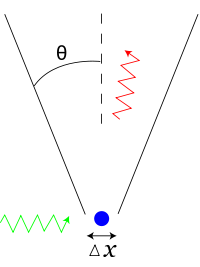Heisenberg's thought experiment for locating an electron (shown in blue) with a high-resolution gamma-ray microscope. The incoming gamma ray (shown in green) is scattered by the electron up into the microscope's aperture angle θ. The scattered gamma ray is shown in red. Classical optics shows that the electron position can be resolved only up to an uncertainty Δx that depends on θ and the wavelength λ of the incoming light.

A key element of quantum mechanics is Heisenberg's uncertainty principle, which forbids the simultaneous measurement of the position and momentum of a particle along the same direction. Remarkably, the uncertainty principle for charged, material particles requires the quantization of light into photons, and even the frequency dependence of the photon's energy and momentum. An elegant illustration is Heisenberg's thought experiment for locating an electron with an ideal microscope. The position of the electron can be determined to within the resolving power of the microscope, which is given by a formula from classical optics$\Delta x \sim \frac{\lambda}{\sin \theta}$

where θ is the aperture angle of the microscope. Thus, the position uncertainty Δx can be made arbitrarily small by reducing the wavelength λ. The momentum of the electron is uncertain, since it received a "kick" Δp from the light scattering from it into the microscope. If light were not quantized into photons, the uncertainty Δp could be made arbitrarily small by reducing the light's intensity. In that case, since the wavelength and intensity of light can be varied independently, one could simultaneously determine the position and momentum to arbitrarily high accuracy, violating the uncertainty principle. By contrast, Einstein's formula for photon momentum preserves the uncertainty principle; since the photon is scattered anywhere within the aperture, the uncertainty of momentum transferred equals$\Delta p \sim p_{\text{photon}} \sin\theta = \frac{h}{\lambda} \sin\theta$

giving the product$\Delta x \Delta p \, \sim \, h$, which is Heisenberg's uncertainty principle. Thus, the entire world is quantized; both matter and fields must obey a consistent set of quantum laws, if either one is to be quantized.

The analogous uncertainty principle for photons forbids the simultaneous measurement of the number n of photons (see Fock state and the Second quantization section below) in an electromagnetic wave and the phase ϕ of that wave

ΔnΔϕ > 1

See coherent state and squeezed coherent state for more details.

Both photons and material particles such as electrons create analogous interference patterns when passing through a double-slit experiment. For photons, this corresponds to the interference of a Maxwell light wave whereas, for material particles, this corresponds to the interference of the Schrödinger wave equation. Although this similarity might suggest that Maxwell's equations are simply Schrödinger's equation for photons, most physicists do not agree. For one thing, they are mathematically different; most obviously, Schrödinger's one equation solves for a complex field, whereas Maxwell's four equations solve for real fields. More generally, the normal concept of a Schrödinger probability wave function cannot be applied to photons. Being massless, they cannot be localized without being destroyed; technically, photons cannot have a position eigenstate$|\mathbf{r} \rangle$, and, thus, the normal Heisenberg uncertainty principle ΔxΔp > h / 2 does not pertain to photons. A few substitute wave functions have been suggested for the photon, but they have not come into general use. Instead, physicists generally accept the second-quantized theory of photons described below, quantum electrodynamics, in which photons are quantized excitations of electromagnetic modes.

## Bose–Einstein model of a photon gas

In 1924, Satyendra Nath Bose derived Planck's law of black-body radiation without using any electromagnetism, but rather a modification of coarse-grained counting of phase space. Einstein showed that this modification is equivalent to assuming that photons are rigorously identical and that it implied a "mysterious non-local interaction", now understood as the requirement for a symmetric quantum mechanical state. This work led to the concept of coherent states and the development of the laser. In the same papers, Einstein extended Bose's formalism to material particles (bosons) and predicted that they would condense into their lowest quantum state at low enough temperatures; this Bose–Einstein condensation was observed experimentally in 1995.

The modern view on this is that photons are, by virtue of their integer spin, bosons (as opposed to fermions with half-integer spin). By the spin-statistics theorem, all bosons obey Bose–Einstein statistics (whereas all fermions obey Fermi-Dirac statistics).

## Stimulated and spontaneous emission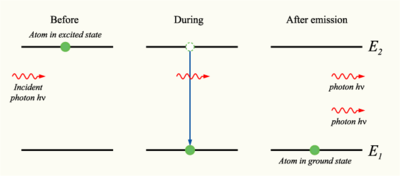Stimulated emission (in which photons "clone" themselves) was predicted by Einstein in his kinetic analysis, and led to the development of the laser. Einstein's derivation inspired further developments in the quantum treatment of light, which led to the statistical interpretation of quantum mechanics.

In 1916, Einstein showed that Planck's radiation law could be derived from a semi-classical, statistical treatment of photons and atoms, which implies a relation between the rates at which atoms emit and absorb photons. The condition follows from the assumption that light is emitted and absorbed by atoms independently, and that the thermal equilibrium is preserved by interaction with atoms. Consider a cavity in thermal equilibrium and filled with electromagnetic radiation and atoms that can emit and absorb that radiation. Thermal equilibrium requires that the energy density ρ(ν) of photons with frequency ν (which is proportional to their number density) is, on average, constant in time; hence, the rate at which photons of any particular frequency are emitted must equal the rate of absorbing them.

Einstein began by postulating simple proportionality relations for the different reaction rates involved. In his model, the rate Rji for a system to absorb a photon of frequency ν and transition from a lower energy Ej to a higher energy Ei is proportional to the number Nj of atoms with energy Ej and to the energy density ρ(ν) of ambient photons with that frequency,$R_{ji} = N_{j} B_{ji} \rho(\nu) \!$

where Bji is the rate constant for absorption. For the reverse process, there are two possibilities: spontaneous emission of a photon, and a return to the lower-energy state that is initiated by the interaction with a passing photon. Following Einstein's approach, the corresponding rate Rij for the emission of photons of frequency ν and transition from a higher energy Ei to a lower energy Ej is$R_{ij} = N_{i} A_{ij} + N_{i} B_{ij} \rho(\nu) \!$

where Aij is the rate constant for emitting a photon spontaneously, and Bij is the rate constant for emitting it in response to ambient photons (induced or stimulated emission). In thermodynamic equilibrium, the number of atoms in state i and that of atoms in state j must, on average, be constant; hence, the rates Rji and Rij must be equal. Also, by arguments analogous to the derivation of Boltzmann statistics, the ratio of Ni and Nj is gi / gjexp (EjEi) / kT), where gi,j are the degeneracy of the state i and that of j, respectively, Ei,j their energies, k the Boltzmann constant and T the system's temperature. From this, it is readily derived that giBij = gjBji and$A_{ij} = \frac{8 \pi h \nu^{3}}{c^{3}} B_{ij}.$

The A and Bs are collectively known as the Einstein coefficients.

Einstein could not fully justify his rate equations, but claimed that it should be possible to calculate the coefficients Aij, Bji and Bij once physicists had obtained "mechanics and electrodynamics modified to accommodate the quantum hypothesis". In fact, in 1926, Paul Dirac derived the Bij rate constants in using a semiclassical approach, and, in 1927, succeeded in deriving all the rate constants from first principles within the framework of quantum theory. Dirac's work was the foundation of quantum electrodynamics, i.e., the quantization of the electromagnetic field itself. Dirac's approach is also called second quantization or quantum field theory; earlier quantum mechanical treatments only treat material particles as quantum mechanical, not the electromagnetic field.

Einstein was troubled by the fact that his theory seemed incomplete, since it did not determine the direction of a spontaneously emitted photon. A probabilistic nature of light-particle motion was first considered by Newton in his treatment of birefringence and, more generally, of the splitting of light beams at interfaces into a transmitted beam and a reflected beam. Newton hypothesized that hidden variables in the light particle determined which path it would follow. Similarly, Einstein hoped for a more complete theory that would leave nothing to chance, beginning his separation from quantum mechanics. Ironically, Max Born's probabilistic interpretation of the wave function was inspired by Einstein's later work searching for a more complete theory.

## Second quantization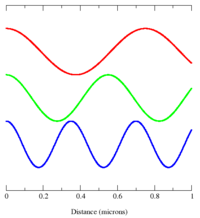Different electromagnetic modes (such as those depicted here) can be treated as independent simple harmonic oscillators. A photon corresponds to a unit of energy E=hν in its electromagnetic mode.

In 1910, Peter Debye derived Planck's law of black-body radiation from a relatively simple assumption. He correctly decomposed the electromagnetic field in a cavity into its Fourier modes, and assumed that the energy in any mode was an integer multiple of hν, where ν is the frequency of the electromagnetic mode. Planck's law of black-body radiation follows immediately as a geometric sum. However, Debye's approach failed to give the correct formula for the energy fluctuations of blackbody radiation, which were derived by Einstein in 1909.

In 1925, Born, Heisenberg and Jordan reinterpreted Debye's concept in a key way. As may be shown classically, the Fourier modes of the electromagnetic field—a complete set of electromagnetic plane waves indexed by their wave vector k and polarization state—are equivalent to a set of uncoupled simple harmonic oscillators. Treated quantum mechanically, the energy levels of such oscillators are known to be E = nhν, where ν is the oscillator frequency. The key new step was to identify an electromagnetic mode with energy E = nhν as a state with n photons, each of energy hν. This approach gives the correct energy fluctuation formula.In quantum field theory, the probability of an event is computed by summing the probability amplitude (a complex number) for all possible ways in which the event can occur, as in the Feynman diagram shown here; the probability equals the square of the modulus of the total amplitude.

Dirac took this one step further. He treated the interaction between a charge and an electromagnetic field as a small perturbation that induces transitions in the photon states, changing the numbers of photons in the modes, while conserving energy and momentum overall. Dirac was able to derive Einstein's Aij and Bij coefficients from first principles, and showed that the Bose–Einstein statistics of photons is a natural consequence of quantizing the electromagnetic field correctly (Bose's reasoning went in the opposite direction; he derived Planck's law of black body radiation by assuming BE statistics). In Dirac's time, it was not yet known that all bosons, including photons, must obey BE statistics.

Dirac's second-order perturbation theory can involve virtual photons, transient intermediate states of the electromagnetic field; the static electric and magnetic interactions are mediated by such virtual photons. In such quantum field theories, the probability amplitude of observable events is calculated by summing over all possible intermediate steps, even ones that are unphysical; hence, virtual photons are not constrained to satisfy E = pc, and may have extra polarization states; depending on the gauge used, virtual photons may have three or four polarization states, instead of the two states of real photons. Although these transient virtual photons can never be observed, they contribute measurably to the probabilities of observable events. Indeed, such second-order and higher-order perturbation calculations can give apparently infinite contributions to the sum. Such unphysical results are corrected for using the technique of renormalization. Other virtual particles may contribute to the summation as well; for example, two photons may interact indirectly through virtual electron-positron pairs. In fact, such photon-photon scattering, as well as electron-photon scattering, is meant to be one of the modes of operations of the planned particle accelerator, the International Linear Collider.

In modern physics notation, the quantum state of the electromagnetic field is written as a Fock state, a tensor product of the states for each electromagnetic mode$|n_{k_0}\rangle\otimes|n_{k_1}\rangle\otimes\dots\otimes|n_{k_n}\rangle\dots$

where$|n_{k_i}\rangle$ represents the state in which$\, n_{k_i}$ photons are in the mode ki. In this notation, the creation of a new photon in mode ki (e.g., emitted from an atomic transition) is written as$|n_{k_i}\rangle \rightarrow |n_{k_i}+1\rangle$. This notation merely expresses the concept of Born, Heisenberg and Jordan described above, and does not add any physics.

## The photon as a gauge boson

The electromagnetic field can be understood as a gauge field, i.e., as a field that results from requiring that a gauge symmetry holds independently at every position in spacetime. For the electromagnetic field, this gauge symmetry is the Abelian U(1) symmetry of a complex number, which reflects the ability to vary the phase of a complex number without affecting observables or real valued functions made from it, such as the energy or the Lagrangian.

The quanta of an Abelian gauge field must be massless, uncharged bosons, as long as the symmetry is not broken; hence, the photon is predicted to be massless, and to have zero electric charge and integer spin. The particular form of the electromagnetic interaction specifies that the photon must have spin ±1; thus, its helicity must be$\pm \hbar$. These two spin components correspond to the classical concepts of right-handed and left-handed circularly polarized light. However, the transient virtual photons of quantum electrodynamics may also adopt unphysical polarization states.

In the prevailing Standard Model of physics, the photon is one of four gauge bosons in the electroweak interaction; the other three are denoted W+, W and Z0 and are responsible for the weak interaction. Unlike the photon, these gauge bosons have mass, owing to a mechanism that breaks their SU(2) gauge symmetry. The unification of the photon with W and Z gauge bosons in the electroweak interaction was accomplished by Sheldon Glashow, Abdus Salam and Steven Weinberg, for which they were awarded the 1979 Nobel Prize in physics. Physicists continue to hypothesize grand unified theories that connect these four gauge bosons with the eight gluon gauge bosons of quantum chromodynamics; however, key predictions of these theories, such as proton decay, have not been observed experimentally.

## Contributions to the mass of a system

The energy of a system that emits a photon is decreased by the energy E of the photon as measured in the rest frame of the emitting system, which may result in a reduction in mass in the amount E / c2. Similarly, the mass of a system that absorbs a photon is increased by a corresponding amount. As an application, the energy balance of nuclear reactions involving photons is commonly written in terms of the masses of the nuclei involved, and terms of the form E / c2 for the gamma photons (and for other relevant energies, such as the recoil energy of nuclei).

This concept is applied in key predictions of quantum electrodynamics (QED, see above). In that theory, the mass of electrons (or, more generally, leptons) is modified by including the mass contributions of virtual photons, in a technique known as renormalization. Such "radiative corrections" contribute to a number of predictions of QED, such as the magnetic dipole moment of leptons, the Lamb shift, and the hyperfine structure of bound lepton pairs, such as muonium and positronium.

Since photons contribute to the stress-energy tensor, they exert a gravitational attraction on other objects, according to the theory of general relativity. Conversely, photons are themselves affected by gravity; their normally straight trajectories may be bent by warped spacetime, as in gravitational lensing, and their frequencies may be lowered by moving to a higher gravitational potential, as in the Pound-Rebka experiment. However, these effects are not specific to photons; exactly the same effects would be predicted for classical electromagnetic waves.

## Photons in matter

Light that travels through transparent matter does so at a lower speed than c, the speed of light in a vacuum. X-rays, on the other hand, usually have a phase velocity above c, as evidenced by total external reflection. In addition, light can also undergo scattering and absorption. There are circumstances in which heat transfer through a material is mostly radiative, involving emission and absorption of photons within it. An example would be in the core of the Sun. Energy can take about a million years to reach the surface. However, this phenomenon is distinct from scattered radiation passing diffusely through matter, as it involves local equilibration between the radiation and the temperature. Thus, the time is how long it takes the energy to be transferred, not the photons themselves. Once in open space, a photon from the Sun takes only 8.3 minutes to reach Earth. The factor by which the speed of light is decreased in a material is called the refractive index of the material. In a classical wave picture, the slowing can be explained by the light inducing electric polarization in the matter, the polarized matter radiating new light, and the new light interfering with the original light wave to form a delayed wave. In a particle picture, the slowing can instead be described as a blending of the photon with quantum excitations of the matter (quasi-particles such as phonons and excitons) to form a polariton; this polariton has a nonzero effective mass, which means that it cannot travel at c.

Alternatively, photons may be viewed as always traveling at c, even in matter, but they have their phase shifted (delayed or advanced) upon interaction with atomic scatters: this modifies their wavelength and momentum, but not speed. A light wave made up of these photons does travel slower than the speed of light. In this view the photons are "bare", and are scattered and phase shifted, while in the view of the preceding paragraph the photons are "dressed" by their interaction with matter, and move without scattering or phase shifting, but at a lower speed.

Light of different frequencies may travel through matter at different speeds; this is called dispersion. In some cases, it can result in extremely slow speeds of light in matter. The effects of photon interactions with other quasi-particles may be observed directly in Raman scattering and Brillouin scattering.

Photons can also be absorbed by nuclei, atoms or molecules, provoking transitions between their energy levels. A classic example is the molecular transition of retinal C20H28O, which is responsible for vision, as discovered in 1958 by Nobel laureate biochemist George Wald and co-workers. The absorption provokes a cis-trans isomerization that, in combination with other such transitions, is transduced into nerve impulses. The absorption of photons can even break chemical bonds, as in the photodissociation of chlorine; this is the subject of photochemistry. Analogously, gamma rays can in some circumstances dissociate atomic nuclei in a process called photodisintegration.

## Technological applications

Photons have many applications in technology. These examples are chosen to illustrate applications of photons per se, rather than general optical devices such as lenses, etc. that could operate under a classical theory of light. The laser is an extremely important application and is discussed above under stimulated emission.

Individual photons can be detected by several methods. The classic photomultiplier tube exploits the photoelectric effect: a photon landing on a metal plate ejects an electron, initiating an ever-amplifying avalanche of electrons. Charge-coupled device chips use a similar effect in semiconductors: an incident photon generates a charge on a microscopic capacitor that can be detected. Other detectors such as Geiger counters use the ability of photons to ionize gas molecules, causing a detectable change in conductivity.

Planck's energy formula E = hν is often used by engineers and chemists in design, both to compute the change in energy resulting from a photon absorption and to predict the frequency of the light emitted for a given energy transition. For example, the emission spectrum of a fluorescent light bulb can be designed using gas molecules with different electronic energy levels and adjusting the typical energy with which an electron hits the gas molecules within the bulb.[Note 5]

Under some conditions, an energy transition can be excited by "two" photons that individually would be insufficient. This allows for higher resolution microscopy, because the sample absorbs energy only in the region where two beams of different colors overlap significantly, which can be made much smaller than the excitation volume of a single beam (see two-photon excitation microscopy). Moreover, these photons cause less damage to the sample, since they are of lower energy.

In some cases, two energy transitions can be coupled so that, as one system absorbs a photon, another nearby system "steals" its energy and re-emits a photon of a different frequency. This is the basis of fluorescence resonance energy transfer, a technique that is used in molecular biology to study the interaction of suitable proteins.

Several different kinds of hardware random number generator involve the detection of single photons. In one example, for each bit in the random sequence that is to be produced, a photon is sent to a beam-splitter. In such a situation, there are two possible outcomes of equal probability. The actual outcome is used to determine whether the next bit in the sequence is "0" or "1".

## Recent research

Much research has been devoted to applications of photons in the field of quantum optics. Photons seem well-suited to be elements of an extremely fast quantum computer, and the quantum entanglement of photons is a focus of research. Nonlinear optical processes are another active research area, with topics such as two-photon absorption, self-phase modulation, modulational instability and optical parametric oscillators. However, such processes generally do not require the assumption of photons per se; they may often be modeled by treating atoms as nonlinear oscillators. The nonlinear process of spontaneous parametric down conversion is often used to produce single-photon states. Finally, photons are essential in some aspects of optical communication, especially for quantum cryptography.[Note 6]

 Doppler shift Light Luminiferous aether Electromagnetic radiation Quantum optics Photonics Phonons Photon polarization Ballistic photon Medipix Photography Laser Advanced Photon Source at Argonne National Laboratory Static forces and virtual-particle exchange Two-photon physics

Wikimedia Foundation. 2010.

### Look at other dictionaries:

• Photon — Photons émis dans le faisceau cohérent d’un laser Propriétés générales Classification Boson Composition Élémentaire Groupe …   Wikipédia en Français

• PHOTON — Le photon est la particule qui décrit les excitations élémentaires du champ électromagnétique quantifié. En théorie quantique, le champ présente, en effet, un double caractère: ondulatoire et corpusculaire. L’aspect ondulatoire se manifeste par… …   Encyclopédie Universelle

• Photon — Klassifikation Elementarteilchen Boson Eichboson Eigenschaften Ladung neutral Masse 0  …   Deutsch Wikipedia

• photon'ic — adjective • • • Main Entry: ↑photon …   Useful english dictionary

• photon — unit of electromagnetic radiation, 1926, from PHOTO (Cf. photo ) light + ON (Cf. on) unit …   Etymology dictionary

• photon —  Photon  Фотон   Квант электромагнитного излучения, нейтральная элементарная частица с нулевой массой покоя и спином 1; переносчик электромагнитного взаимодействия между заряженными частицами. Может находиться только в двух спиновых состояниях …   Толковый англо-русский словарь по нанотехнологии. - М.

• photon — ► NOUN Physics ▪ a particle representing a quantum of light or other electromagnetic radiation. DERIVATIVES photonic adjective …   English terms dictionary

• photon — ☆ photon [fō′tän΄ ] n. [ PHOT(O) + (ELECTR)ON] 1. Particle Physics a subatomic particle, having energy and momentum but no mass or electric charge, that is the quantum unit of electromagnetic radiation, including light: see also CLASSON 2. a unit …   English World dictionary

• photon — /foh ton/, n. a quantum of electromagnetic radiation, usually considered as an elementary particle that is its own antiparticle and that has zero rest mass and charge and a spin of one. Symbolically represented by the small greek letter Gamma.… …   Universalium

• Photon — fotonas statusas T sritis Standartizacija ir metrologija apibrėžtis Elementarioji elektromagnetinės spinduliuotės dalelė, kurios masė lygi nuliui. Jos energija: E = hν; čia h – Planko konstanta, ν – spinduliuotės dažnis. Fotonai juda šviesos… …   Penkiakalbis aiškinamasis metrologijos terminų žodynas